15.4 C
New York
Tuesday, September 19, 2023
HomeML & AITop 50 Frequently Asked Machine Learning Interview Questions and Answers

At present, machine learning, artificial intelligence, and data science are the most booming factor to bring the next revolution in this industrial and technology-driven world. Therefore, there are a significant number of opportunities that are waiting for fresh graduate data scientists and machine learning developers to apply their specific knowledge in a particular domain. However, it’s not that easy as you are thinking. The interview procedure that you will have to go through will definitely be very challenging, and you will have hard competitors. Moreover, your skill will be tested in different ways, i.e., technical and programming skills, problem-solving skills, and your ability to apply machine learning techniques efficiently and effectively, and your overall knowledge about machine learning. To help you with your upcoming interview, in this post, we have listed frequently asked machine learning interview questions.

### Machine Learning Interview Questions & Answers

Traditionally, to recruit a machine learning developer, several types of machine learning interview questions are asked. Firstly, some basic machine learning questions are asked. Then, machine learning algorithms, their comparisons, benefits, and drawbacks are asked. Finally, the problem-solving skill using these algorithms and techniques are examined. Here, we outlined interview questions on machine learning to guide your interview journey.

#### Q-1: Explain the Concept of Machine Learning like a School going, Student.

The concept of machine learning is quite simple and easy to understand. It’s like how a baby learns to walk. Every time the baby falls, and he gradually realizes that he should keep his leg straight to move. When he falls, he feels pain. But, the baby learns not to walk like that again. Sometimes the baby seeks support to walk. This is the way how a machine develops gradually. First, we develop a prototype. Then we continuously improve it with the requirements.

#### Q-2: Explain what Machine Learning is all about?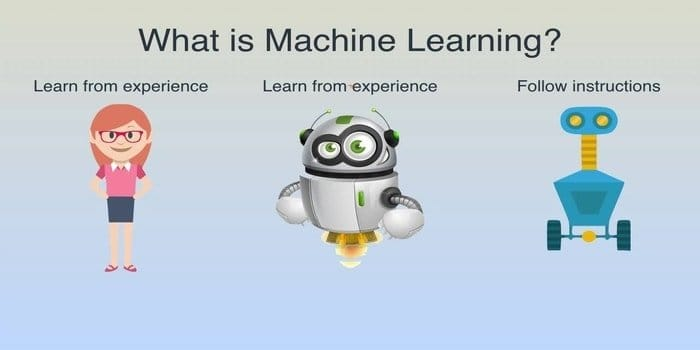Machine Learning is the study of algorithms that develop a system that is so intelligent that it can act just like a human being. It builds a machine or device in such a way that its ability to learn without any explicit instructions.  The phenomena of machine learning make a machine able to learn, identify patterns, and make a decision automatically.

#### Q-3: Core Difference between Supervised and Unsupervised Machine Learning.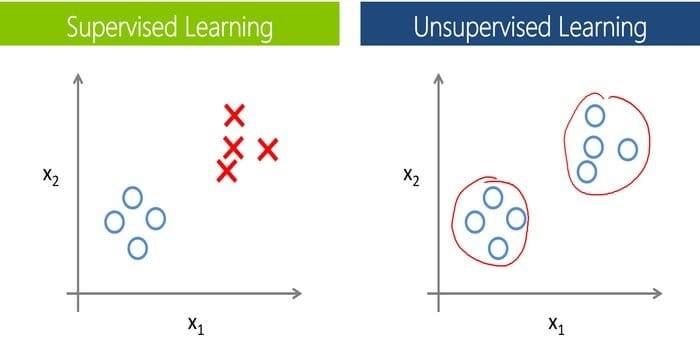This question is one of the most common interview questions on machine learning. Also, this is one of the basic ml questions. To train machines and models, labeled data is required in supervised learning. That means a certain amount of data is already tagged with the actual output. Now, as a major difference, we do not need labeled data in unsupervised learning.

#### Q-4: How does Deep Learning differ from Machine Learning?This type of question is very common in any deep learning interview questions and often asked by the interviewers to justify candidates. We can incorporate Deep learning into machine learning and following that, machine learning into artificial intelligence, thereby connecting all three. This is possible only because each is a subcategory of the other. Therefore we can also say that it is an advanced level of machine learning. But nonetheless, the interpretability of deep learning is 10 times faster than machine learning.

#### Q-5: Difference between Data Mining and Machine Learning.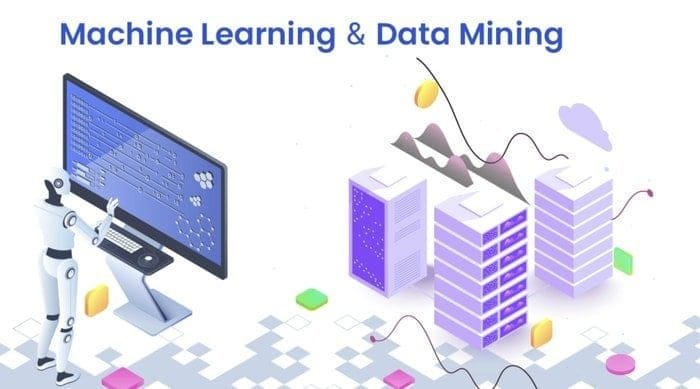In any ML interview questions, this sort of question is very common. Also, if your basic is clear, then you can answer this type of question effortlessly. It would be wrong to say that machine learning and data mining are completely different because they have quite a few similarities, but then again, few fine lines make a difference both of them.

The core difference is in their meaning; the term data mining corresponds to the extraction of patterns by mining data, and the term machine learning means making an autonomous machine. The main objective of data mining is using unstructured data to find out the hidden patterns that can be used for the future.

On the other hand, the purpose of machine learning is to build an intelligent machine that can learn independently according to the environment. To learn in detail, you may go through our data mining vs. machine learning post.

#### Q-6: Differences between Artificial Intelligence and Machine Learning?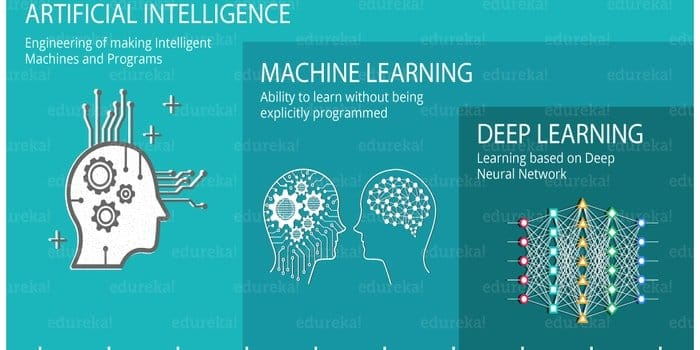Almost in all interview questions on machine learning or artificial intelligence, it is a common question because most of the candidates think that both are the same thing. Although there is crystal clear distinction between them, it is often the case when artificial intelligence and machine learning are used in place of one another and this exactly the root of the confusion.

Artificial intelligence is a broader prospect than machine learning. Artificial intelligence mimics the cognitive functions of the human brain. The purpose of AI is to carry out a task in an intelligent manner based on algorithms. On the other hand, machine learning is a subclass of artificial intelligence. To develop an autonomous machine in such a way so that it can learn without being explicitly programmed is the goal of machine learning.

#### Q-7: Mention Five Popular Machine Learning Algorithms.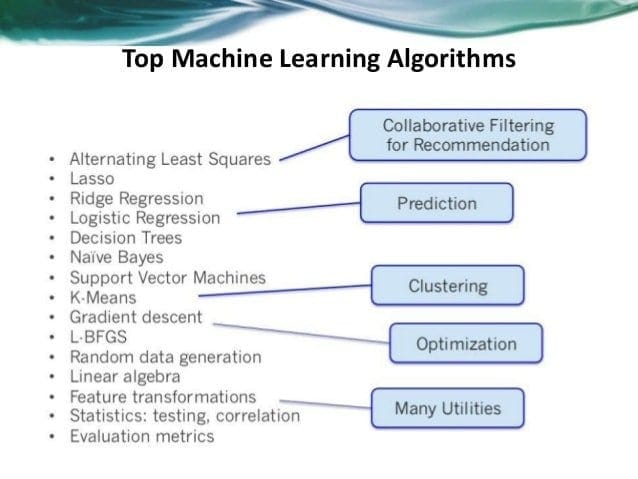If someone wants to develop an artificial intelligence and machine learning project, you have several options for choosing machine learning algorithms. Anyone can pick the appropriate algorithm easily according to their system demand. The five machine learning algorithms are Naive Bayes, Support Vector Machine, Decision Tree, K- Nearest Neighbor (KNN), and K- means. For details, you may also read our previous article on machine learning algorithms.

#### Q-8: Make a Comparison between Machine Learning and Big Data.

If you are a fresh job candidate, then this sort of question is quite common as ML interview questions. By asking this type of question, the interviewer tries to understand the in-depth of your knowledge of machine learning. The main difference between big data and machine learning lies in their definition or purpose.

Big data is the approach of collecting and analyzing a large volume of datasets (called Big Data). The purpose of big data is to discover useful hidden patterns from a large volume of data which is helpful for organizations. On the contrary, machine learning is the study of making an intelligent device that can perform any task without explicit instructions.

A significant advantage of a decision tree is that it traces each possible outcome of a decision into a deduction, and it does this by considering all outcomes. It creates a broad analysis of the consequences along each branch and identifies the decision nodes that need further analysis.

One of the primary disadvantages of a decision tree is their instability, which means that the structure of the optimal decision tree will be highly affected by only a minor change in the data. Sometimes the values are not known, and the outcomes are very closely linked, and this causes calculations to become very complex.

#### Q-10: Describe the Comparison between Inductive Machine Learning and Deductive Machine Learning.

This type of question is pretty commonly asked in an ML interview. Deductive machine learning studies algorithms for learning knowledge that is capable of being proved in some way. To speed up problem solvers, these methods are typically used, by adding knowledge to them deductively using existing knowledge. This will result in faster solutions.

If you look at it from the viewpoint of inductive learning, you will see that the problem will be to estimate the function (f) from a certain input sample (x) and an output sample (f(x)) that will be given to you. More specifically, you have to generalize from the samples, and this is where the problem arises. To make the mapping useful is another issue that you will have to face so that it is easier to estimate the output for new samples in the future.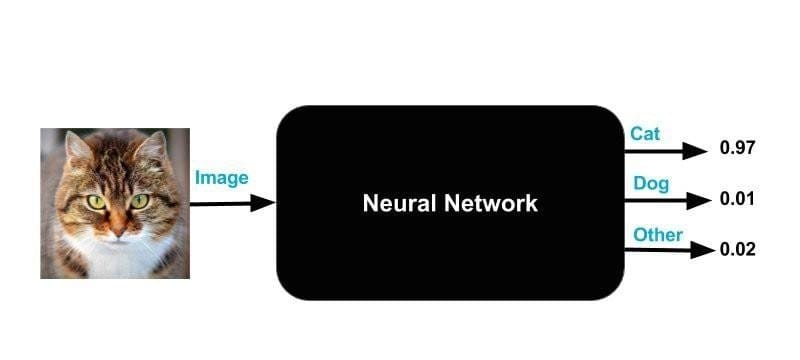This is a very important machine learning interview question and also serves as a primary question among all your deep learning interview questions. The main advantages of neural networks are that it can handle large amounts of data sets; they can implicitly detect complex nonlinear relationships between dependent and independent variables. Neural networks can outweigh almost every other machine learning algorithms, although some disadvantages are bound to stay.

Such as the black-box nature is one of the best-known disadvantages of neural networks. To simplify it further, you will not even know how or why your NN came up with a certain output whenever it gives you one.

#### Q-12: Steps Needed to Choose the Appropriate Machine Learning Algorithm for your Classification problem.

Firstly, you need to have a clear picture of your data, your constraints, and your problems before heading towards different machine learning algorithms. Secondly, you have to understand which type and kind of data you have because it plays a primary role in deciding which algorithm you have to use.

Following this step is the data categorization step, which is a two-step process – categorization by input and categorization by output. The next step is to understand your constraints; that is, what is your data storage capacity? How fast the prediction has to be? etc.

Finally, find the available machine learning algorithms and implement them wisely. Along with that, also try to optimize the hyperparameters which can be done in three ways – grid search, random search, and Bayesian optimization.

#### Q-13: Can you Explain the Terms “Training Set” and “Test Set”?

To train models for performing various actions, the training set is used in machine learning. It helps to train the machines to work automatically with the help of various API and algorithms. By fitting the particular model into the training set, this set is processed, and after that, this fitted model is used to predict the responses for the observations in the validation set, thereby linking the two.

After the machine learning program has been trained on an initial training data-set, it is then put on test in the second dataset, which is the test set.

#### Q-14: What is “Overfitting”?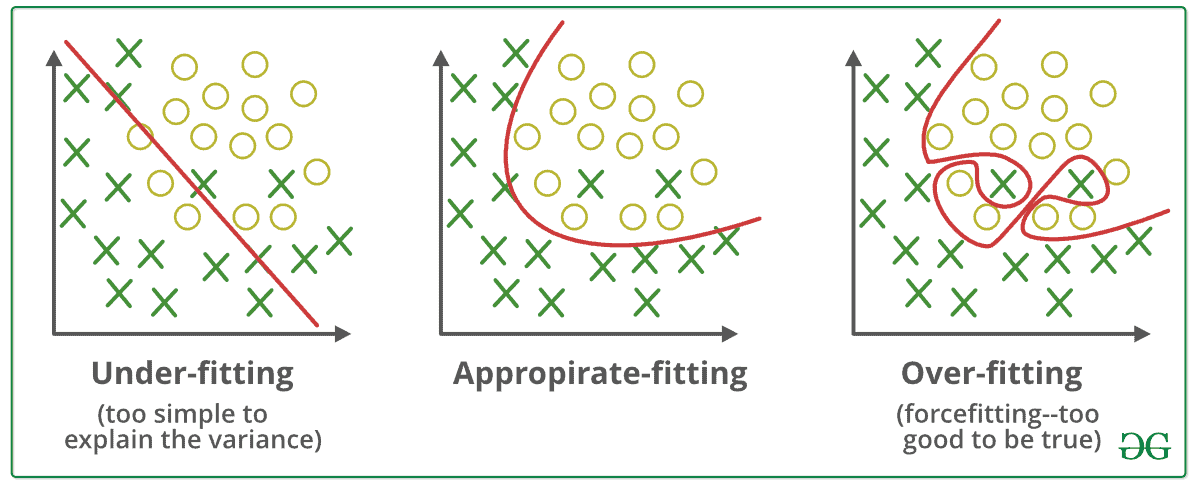In machine learning, a model that models the training data too well is referred to as overfitting. This occurs when a model acquires the details and noises in the training set and takes it as a piece of important information for the new data. This negatively impacts the enactment of the model as it picks up these random fluctuations or sounds as necessary concepts for the new model, whereas it doesn’t even apply to it.

#### Q-15: Define a Hash Table.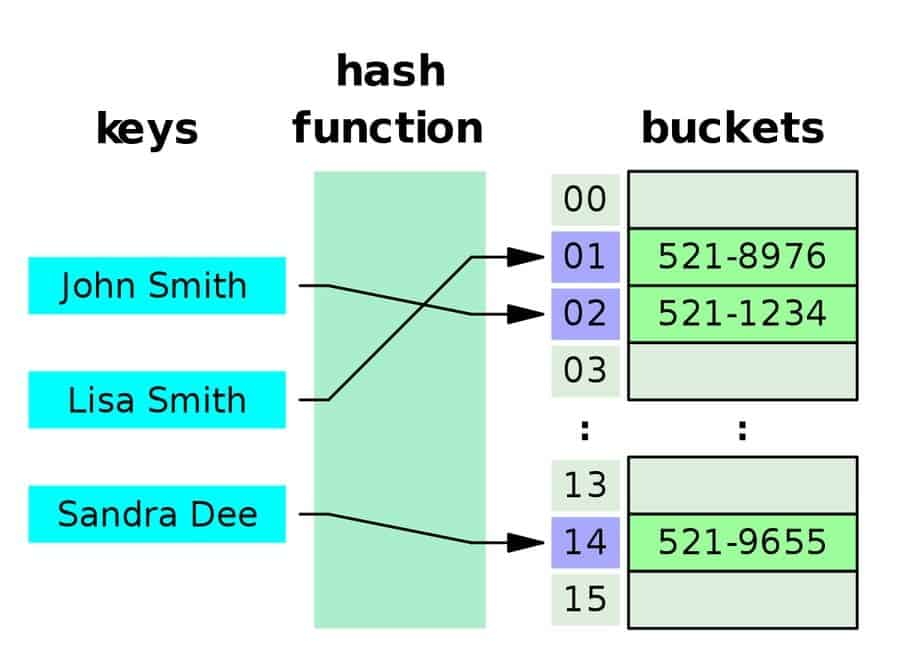Hash table is a data structure that piles data in an ordered arrangement where each data has its unique index value. In other words, data is stored in an associative manner. This means that the size of the data structure doesn’t even matter and thus, the insert and search operations are very quick to operate in this data structure. To compute an index into an array of slots, a hash table uses a hash index, and from there the desired value can be found.

#### Q-16: Describe the Use of Gradient Descent.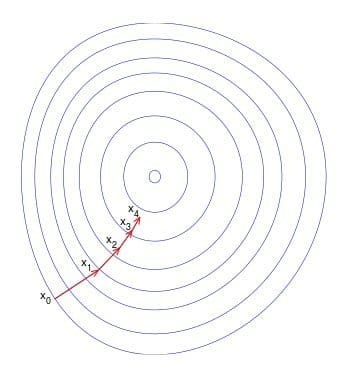This is quite an occurring question for both machine learning interviews as well as a deep learning interview questions. Gradient descent is used to update the parameters of your model in machine learning. It is an optimization algorithm that can minimize a function to its simplest form.

It is usually used in linear regression, and this is because of the computational complexity. In some cases, it is cheaper and faster to find the solution of a function using gradient descent, and thereby, it saves a lot of time in calculations.

#### Q-17: Define Bucketing in terms of Machine Learning.

Bucketing is a process in machine learning which is used to convert a feature into multiple binary features called buckets or bins, and this is typically based on value range.

For example, you can chop ranges of temperatures into discrete bins instead of representing temperature as a single continuous floating-point feature. For example temperatures between 0-15 degrees can be placed into one bucket, 15.1-30 degrees can be put into another bucket and so on.

#### Q-18: Narrate Backpropagation in Machine Learning.

A very important question for your machine learning interview. Backpropagation is the algorithm for computing artificial neural networks (ANN). It is used by the gradient descent optimization that exploits the chain rule. By calculating the gradient of the loss function, the weight of the neurons is adjusted to a certain value. To train a multi-layered neural network is the prime motivation of backpropagation so that it can learn the appropriate internal demonstrations. This will help them learn to map any input to its respective output arbitrarily.

#### Q-19: What’s the Confusion Matrix?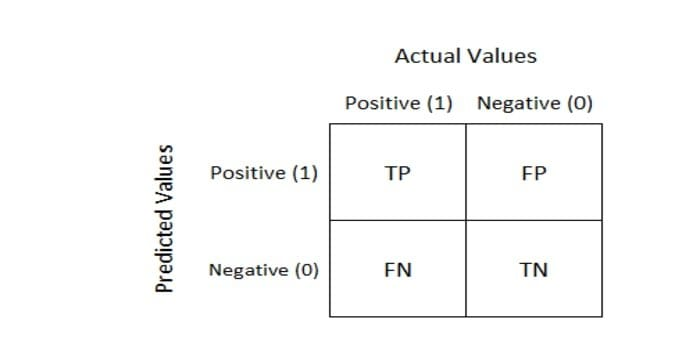This question is often listed in interview questions on machine learning. So whenever we want to measure the performance of a machine learning classification problem, we use a Confusion Matrix. The output can be two or more classes. The table consists of four different combinations of predicted and actual values.

#### Q-20: Differentiate Classification and Regression.

Let us get this clear in our heads that Classification and Regression are categorized under the same hat of supervised machine learning. The focal difference between them is that the output variable for regression is numerical or continuous and that for classification is categorical or discrete, which is in the form an integer value.

To set up as an example, classifying an email as spam or non-spam is an example of a classification problem and predicting the price of a stock over some time is an example of a regression problem.

#### Q-21: Define A/B Testing.A/B testing is an experiment that is randomly done using two variants A and B, and it is done to compare two versions of a webpage to figure out the better performing variation for a given conversion goal.

#### Q-22: Define the Sigmoid Function.

This question is often enlisted in machine learning interview questions. The sigmoid function has a characteristic “S-shape”; it is a mathematical function that is bounded and differentiable. It is a real function that is definite for all real input values and has a non-negative, which is ranging from 0-1, the derivative at each point.#### Q-23: What is the Convex Function?

This question is very often asked in machine learning interview. A convex function is a continuous function, and the value of the midpoint at every interval in its given domain is less than the numerical mean of the values at the two ends of the interval.

#### Q-24: List some Key Business Metrics that are Useful in Machine Learning.

• Confusion matrix
• Accuracy metric
• Recall / Sensitivity metric
• Precision metric
• Root mean square error

#### Q-25: How can you handle Missing Data to Develop a Model?

There are several methods in which you can handle missing data while developing a model.

Listwise deletion: You can delete all the data from a given participant with missing values using pair-wise or listwise deletion. This method is used for data that are missed randomly.

Average imputation: You can take the average value of the responses from the other participants to fill up the missing value.

Common – point imputation: You can take the middle point or the most commonly chosen value for a rating scale.

#### Q-26: How much Data will you use in your Training set, Validation, and Test set?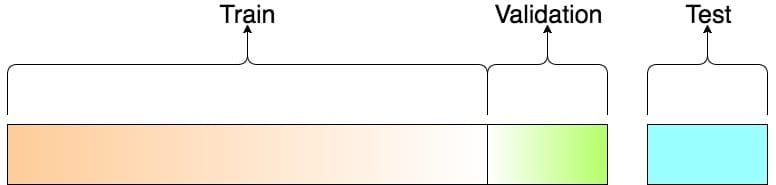This is very important as machine learning interview questions. There needs to be a balance while choosing data for your training set, validation set, and test set.

If the training set is made too small, then the actual parameters will have high variance and in the same way, if the test set is made too small, then there are chances of unreliable estimation of model performances. Generally, we can divide the train/test according to the ratio of 80:20, respectively. The training set can then be further divided into the validation set.

#### Q-27: Mention some Feature Extraction Techniques for Dimensionality Reduction.

• Independent Component Analysis
• Isomap
• Kernel PCA
• Latent Semantic Analysis
• Partial Least Squares
• Semidefinite Embedding
• Autoencoder

#### Q-28: Where you can Apply Classification Machine Learning Algorithms?

Classification machine learning algorithms can be utilized for grouping information completely, positioning pages, and ordering importance scores. Some other uses include identifying risk factor related to diseases and planning preventive measures against them

It is used in weather forecasting applications to predict the weather conditions and also in voting applications to understand whether voters will vote for a particular candidate or not.

On the industrial side, classification machine learning algorithms have some very useful applications, that is, finding out whether a loan applicant is at low-risk or high-risk and also in automobile engines for predicting the failure of mechanical parts and also predicting social media share scores and performance scores.

#### Q-29: Define the F1 Score in Terms of Artificial Intelligence Machine Learning.This question is a very common one in AI and ML interviews. The F1 score is defined as the harmonic weighted average (mean) of precision and recall, and it is used to measure the performance of an individual statistically.

As already described, the F1 score is an evaluation metric, and it is used to express the performance of a machine learning model by giving combined information about the precision and recall of a model. This method is usually used when we want to compare two or more machine learning algorithms for the same data.

This is pretty common in ML interview questions. The Bias – Variance tradeoff is the property that we need to understand for predicting models. To make a target function easier to work, a model makes simplifying assumptions that are known as bias. By using different training data, the amount of change that would cause the target function is known as Variance.

Low bias, along with low variance is the best possible outcome, and that is why to attain this is the ultimate goal of any unsupervised machine learning algorithm because it then provides the best prediction performance.

#### Q-31: Why can’t we Use Manhattan Distance in K-means or KNN?

Manhattan distance is used to calculate the distance between two data points in a grid-like path. This method cannot be used in KNN or k-means because the number of iterations in Manhattan’s distance is less due to the direct proportionality of computational time complexity to the number of iterations.

#### Q-32: How can a Decision Tree be Pruned?

This question is something that you will not want to miss out on as it is equally important for both machine learning interview questions as well as artificial intelligence interview questions. Pruning is done to reduce the complexity and increase the predictive accuracy of a decision tree.

With reduced error pruning and cost complexity pruning technique, it can be done in a bottom-up and top-down manner. The reduced error pruning technique is very uncomplicated; it just replaces each node, and if the predictive accuracy does not decrease, it continues pruning.

#### Q-33: When does a Developer use Classification in place of Regression?

As a fresh graduate, you should know the proper area of use of each of these, and therefore, it stands as a model question in machine learning interviews. Classification is identifying group membership, while the regression technique involves predicting a response.

Both these techniques are related to prediction, but a classification algorithm predicts a continuous value, and this value is in the form of a probability for a class label. Therefore, a developer should use a classification algorithm when there is a task of predicting a discrete label class.

#### Q-34: Which one is essential: Model Accuracy or Model Performance?

Model accuracy is the most important characteristic of a machine learning model and thereby obviously more important than model performance; it solely depends on the training data.

The reason behind this importance is that the accuracy of the model has to be carefully built during the model training process, but model performance can always be improved by parallelizing over the scored assets and also by using distributed computing.

#### Q-35: Define a Fourier Transform.

The Fourier transform is a mathematical function that takes time as the input and decomposes a waveform into the frequencies that make it up. The output/result produced by it is a complex-valued function of frequency. If we find out the absolute value of a Fourier transform, we will get the value of the frequency that is present in the original function.

#### Q-36: Differentiate KNN vs. K-means Clustering.

Before we dive into their difference, we first need to know what they are and where their main contrast is. Classification is done by KNN, which is a supervised learning algorithm, whereas clustering is the job of K-means, and this is an unsupervised learning algorithm.

KNN needs labeled points, and K-means does not, and this stands as a sharp difference between them. A set of unlabeled points and a threshold is the only requirement for K-means clustering. Due to this lack of unlabeled points, k – means clustering is an unsupervised algorithm.

#### Q-37: Define Bayes’ Theorem. Focus on its Importance in a Machine Learning Context.

Bayes’ Theorem gives us the probability that an event will take place based on preceding knowledge that is eventually related to the event. Machine learning is a set of methods for creating models that predict something about the world, and this is done by learning those models from the given data.

Thus, Bayes Theorem allows us to encrypt our prior opinions as to how the models should look like, independent of the data provided. When we do not have as much information about the models, this method becomes quite convenient for us at that time.

#### Q-38: Differentiate Covariance vs. Correlation.

Covariance is a measure of how much two random variables can change, whereas correlation is a measure of how related two variables are to each other. Therefore, covariance is a measure of correlation, and correlation is a scaled version of covariance.

If there is any change in the scale, it does not have any effect on the correlation, but it does influence the covariance. Another difference is in their values, that is, the values of covariance lie between (–) infinity to (+) infinity, whereas the values of the correlation lie between -1 and +1.

#### Q-39: What’s the Relationship between True Positive Rate and Recall?The True positive rate in machine learning is the percentage of the positives that have been properly acknowledged, and recall is just the count of the results that have been correctly identified and are relevant. Therefore, they are the same things, just having different names. It is also known as sensitivity.

#### Q-40: Why is “Naive” Bayes called Naive?

This is a question that you won’t want to miss out on as this is also an important question for your artificial intelligence job interviews. The Naïve Bayes is a classifier, and it assumes that, when the class variable is given, the presence or absence of a particular feature does not affect and is thereby independent of the presence or absence of any other feature. Therefore we call it “naïve” because the assumptions that it makes are not always correct.

#### Q-41: Explain the terms Recall and Precision.

This is just another question that is equally important for deep learning job interviews as well as ml interview questions. Precision, in machine learning, is the fraction of relevant cases among the preferred or chosen cases, whereas recall, is the portion of relevant instances that have been selected over the total amount of relevant instances.

#### Q-42.: Define the ROC Curve and Explain its Uses in machine learning.ROC curve, short for receiver operating characteristic curve, is a graph that plots the True Positive Rate against the False Positive Rate, and it mainly evaluates the diagnostic abilities of classification models. In other words, it can be used to find out the accuracy of classifiers.

In machine learning, a ROC curve is used to visualize the performance of a binary classifier system by calculating the area under the curve; basically, it gives us the trade-off between the TPR and FPR as the discrimination threshold of the classifier is varied.

The area under the curve tells us whether it is a good classifier or not and the score usually varies from 0.5 – 1, where a value of 0.5 indicates a bad classifier and a value of 1 indicates an excellent classifier.

#### Q-43: Differentiate between Type I and Type II Error.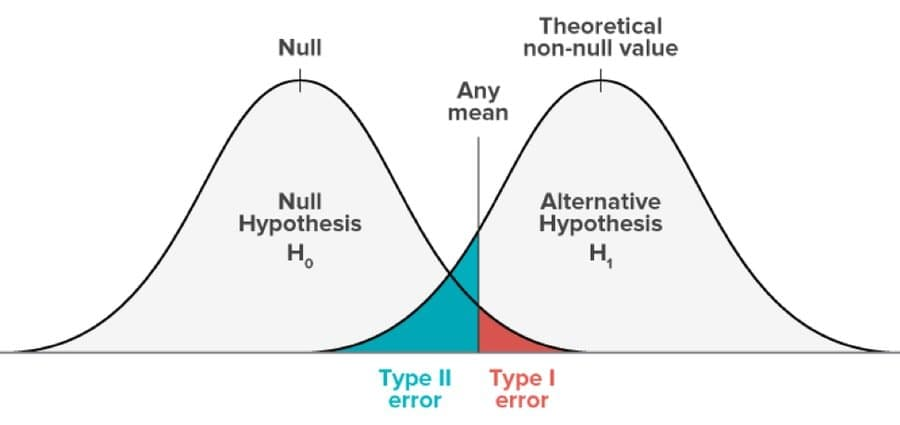This type of error occurs while hypothesis testing is done. This testing is done to decide whether a particular assertion made on a population of data is right or wrong. Type I error takes place when a hypothesis that should be accepted is declined, and Type II error arises when a hypothesis is wrong and should be rejected, but it gets accepted.

Type I error is equivalent to false-positive, and Type II error is equivalent to a false negative. In Type I error, the probability of committing error equals the level of significance of it, whereas, in type II, it equals the influence of test.

#### Q-44: List some Tools for Parallelizing Machine Learning Algorithms.

Although this question may seem very easy, make sure not to skip this one because it is also very closely related to artificial intelligence and thereby, AI interview questions. Almost all machine learning algorithms are easy to serialize. Some of the basic tools for parallelizing are Matlab, Weka, R, Octave, or the Python-based sci-kit learn.

#### Q-45: Define Prior Probability, Likelihood, and Marginal Likelihood in terms of Naive Bayes Machine Learning Algorithm?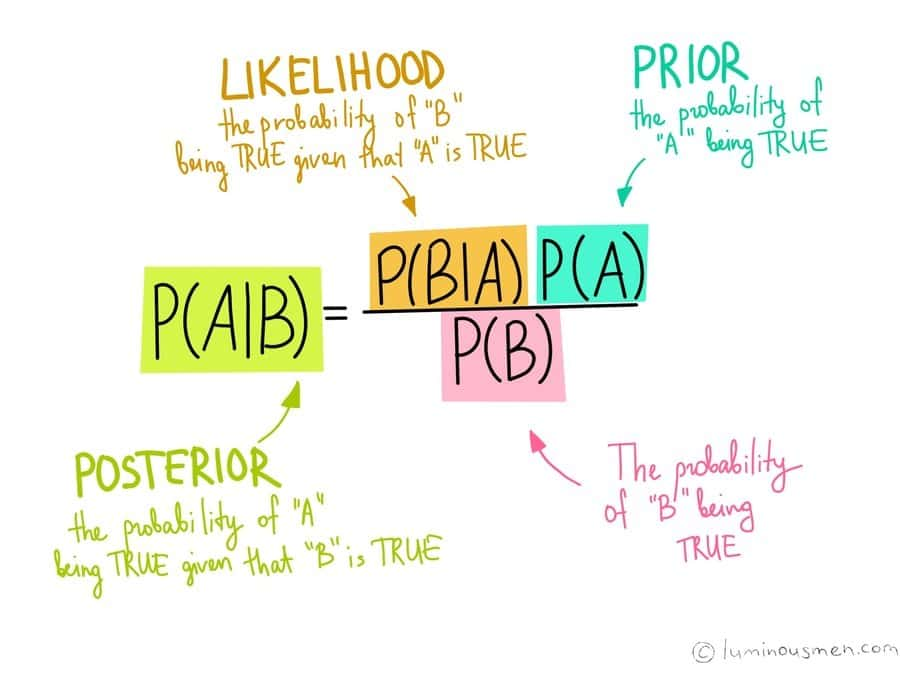Although it is a very common question in machine learning interviews, it sometimes leaves the candidate quite blank in front of the judges. Well, a prior probability is principally the output that is computed before collecting any kind of new data; it is solely done based on the observations previously made.

Now, likelihood in the Naïve Bayes machine learning algorithm is the probability that an event that has already taken place, will have a certain outcome and this outcome is solely based on old events that have occurred. The marginal likelihood is referred to as model evidence in Naïve Bayes machine learning algorithms.

#### Q-46: How do you Measure the Correlation between Continuous and Categorical Variables?

Before heading towards the answer to this question, you first need to understand what correlation means. Well, correlation is the measure of how closely related two variables are linear.

As we know, categorical variables contain a restricted amount of categories or discrete groups whereas, and continuous variables contain an infinite number of values between any two values which can be numeric or date/time.

Therefore, to measure the correlation between continuous and categorical variables, the categorical variable needs to have less or equal to two levels and never more than that. This is because, if it has three or four variables, the whole concept of correlation breaks down.

#### Q-47: Define the most Frequent Metric to Evaluate Model Accuracy.

Classification accuracy is the most frequently used metric to evaluate our model accuracy. The proportion of correct predictions to the total number of prediction samples is the classification accuracy. If there are an unequal number of samples in each class, then this metric cannot function properly. Rather, it works best with an equal number of samples in a class.

#### Q-48: How is Image Processing related to Machine Learning?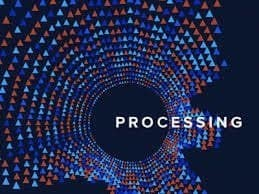Now, this topic is undoubtedly one of the most important topics and so expect this question as a must be one in your machine learning interview questions. It’s not only important for machine learning but also other sectors such as deep learning interview questions and artificial intelligence interview questions.

A very brief description of image processing would be that it is a 2-D signal processing. Now if we want to incorporate image processing into machine learning, we would have to view it as image processing working as a pre-processing step to computer vision. We can use image processing to enhance or eradicate images used in machine learning models or architectures, and this helps to develop the performance of the machine learning algorithms.

#### Q-49: When should we use SVM?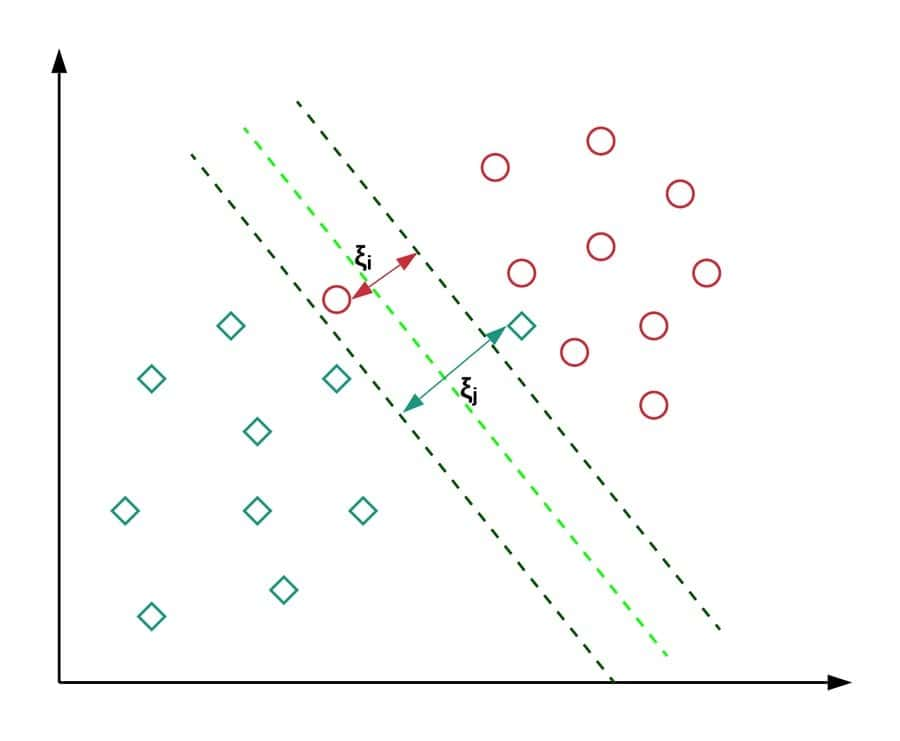SVM stands for support vector machines; it is a supervised machine learning algorithm and can be used to solve problems related to classification and regression. In classification, it is used to differentiate between several groups or classes, and in regression, it is used to obtain a mathematical model that would be able to predict things. One very big advantage of using SVM is that it can be used in both linear and non-linear problems.

#### Q-50: Is Rotation necessary in PCA?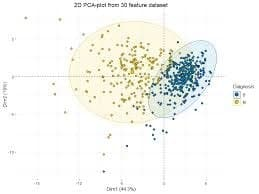PCA is the short form of Principal component analysis. As much as it is important for machine learning interviews, it is equally important in artificial intelligence, and thereby, you might get this question asked in your artificial intelligence interview questions. Rotation is not necessary for PCA, but when used, it optimizes the computation process and makes the interpretation easy.

### Ending Thoughts

Machine learning is a vast area, and also it is incorporated with many other areas like data science, artificial intelligence, big data, data mining, and so forth. Therefore, any tricky and complicated ML interview questions can be asked to examine your knowledge of machine learning. So you have always to keep your skill up-to-date and furnish. You have to learn and practice more and more machine learning techniques scrupulously.Mehedi Hasan
Mehedi Hasan is a passionate enthusiast for technology. He admires all things tech and loves to help others understand the fundamentals of Linux, servers, networking, and computer security in an understandable way without overwhelming beginners. His articles are carefully crafted with this goal in mind - making complex topics more accessible.